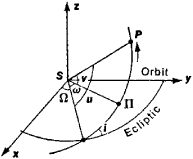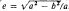# Orbit of a Celestial Body

The following article is from The Great Soviet Encyclopedia (1979). It might be outdated or ideologically biased.

## Orbit of a Celestial Body

the trajectory along which a celestial body moves. The shape of the orbits and the velocity with which the celestial body moves along the orbit are determined by many factors, examples of which are the force of gravity, the force of light pressure, electromagnetic forces, the resistance of the medium in which the motion occurs, tidal forces, and, in the case of the motion of the nucleus of a comet, reactive forces. The force of universal gravitation is of decisive importance in the motion of the planets, comets, planetary satellites, and the sun and the other stars in the Milky Way Galaxy. In the powered segments of the orbits of spacecraft, the determining factor is the reactive force of the propulsion system together with gravitational forces.

The orientation of an orbit in space, the size and shape of the orbit, and the position of the celestial body in the orbit are determined by quantities, or parameters, called the elements of the orbit. The orbital elements of planets, comets, and moons are determined from astronomical observations in three stages. (1) The elements of a preliminary orbit are computed without considering perturbations; that is, the two-body problem is solved. For this purpose it is sufficient in most cases to have three observations—that is, the coordinates of three points on the celestial sphere—of the celestial body, which may be, for example, an asteroid; the observations should have been made over a period of several days or weeks. (2) The preliminary orbit is refined—that is, more precise values for the elements of the orbit are calculated—through longer observations. (3) There is computed a final orbit, which best agrees with all the available observations.

Extensive observations already exist for many bodies in the solar system, including the planets, the moon, and certain planetary satellites. Analytical and numerical methods of celestial mechanics are used to calculate a final orbit from the observations, a process referred to as constructing a theory of the motion of a celestial body.

In the first stage, the orbit takes the form of a conic section, such as an ellipse, a parabola, or a hyperbola. The other, or central, body is located at a focus of the conic. Such orbits are called unperturbed, or Keplerian since a celestial body moves along them according to Kepler’s laws. Six elements define the heliocentric unperturbed orbit of a celestial body P (Figure 1).

(1) The inclination i of the orbit to the plane of the ecliptic. The inclination may have any value from 0° to 180°. It is regarded as less than 90° if the planet appears to an observer located at the north ecliptic pole to move in a counterclockwise direction and greater than 90° if in the opposite direction.Figure 1. Elliptical orbit of a planet in space: S is the sun, P the planet, and Π the orbit’s perihelion. The axis Sx is directed toward the vernal equinox.

(2) The longitude of the ascending node ft, that is, the heliocentric longitude of the point at which the planet intersects the ecliptic in crossing from the southern to the northern hemisphere. The longitude of the ascending node can assume values from 0° to 360°.

(3) The semimajor axis a of the orbit. Sometimes the mean daily motion n, which is the arc of the orbit traversed by the body in a day, is taken as an orbital element instead of a.

(4) The eccentricity e of the orbit. If b is the semiminor axis, then. Sometimes the eccentricity is replaced by the angle of eccentricity ɸ, which is defined by the relation sin ɸ = e.

(5) The angular distance between perihelion and the ascending node, or the perihelion argument ω. The element ω, which can have any value from 0° to 360°, is the heliocentric angle between the orbit’s ascending node and the orbit’s perihelion and is measured in the orbital plane in the direction of the planet’s motion. The longitude of perihelion π= Ω + ω is sometimes used instead of ω.

(6) The element of time, that is, the epoch, or date, at which the planet is located at a specific point in its orbit. This element might be, for example, the moment t at which the planet passes through perihelion. The position of a planet in its orbit is determined by the latitude argument u, which is the angular distance between the planet and the ascending node, or by the true anomaly v, which is the angular distance of the planet from perihelion. The latitude argument varies from 0° to 360° in the direction of the planet’s motion.

Analogous elements define the orbits of comets, the moon, natural satellites of the planets, the components of binary stars, the sun, and other celestial bodies. The term “perihelion,” however, is replaced in these cases by the more general term “peri-center” or by such specialized terms as “perigee,” for the moon moving in a geocentric orbit, and “periastron,” for the components of binary stars.

The task of improving, or refining, a preliminary orbit with the aid of additional observations is accomplished through successive approximations. The greater the interval of time covered by the observations, the more reliably determined are the elements of the improved orbit. In reality, not only gravitational forces but also other perturbing forces are at work, and the motion of a celestial body does not conform to Kepler’s laws. The deviation of the motion from the unperturbed case, however, is not great, and the motion can therefore be described by the formulas of unperturbed motion; the orbital elements are assumed not to maintain constant values but to change over time. Thus, an actual orbit can be regarded as the envelope of a family of continuously changing Keplerian orbits. Moreover, at each moment of time, the position and velocity of the celestial body in the actual orbit coincide with the values for position and velocity the body would have if it were moving in a Keplerian orbit with elements computed specifically for that moment. The orbit determined by this method for a given moment of time t is called the osculating orbit, and the moment t the epoch of osculation. The osculating orbit continuously changes its shape and position in space.

The method for determining an initial parabolic orbit was worked out by H. Olbers in 1797, and the elliptical case was worked out by K. Gauss in 1809. Much work in the 19th and 20th centuries has been devoted to the refinement of orbits and the determination of final orbits. The orbital elements of the planets, asteroids, and comets are regularly given in astronomical yearbooks and almanacs and other publications.

The classical methods of celestial mechanics have been successfully applied to the computation of the orbits of artificial earth satellites. In this case, scientists take into account secular changes in the orbit’s semimajor axis, in the longitude of the ascending node, and in the latitude argument that are due to atmospheric drag, the earth’s nonspherical shape, and in certain cases even solar light pressure. Radio, radar, and laser longdistance methods of observing satellites permit direct determination of the satellites’ distances and radial velocities. Similar observational methods have been applied to natural celestial bodies, for example, the radiolocation of Venus and Mars and the laser location of the moon. The mid-20th century thus has seen the development of new methods for determining orbits, methods that are especially adapted to observations made with modern instruments.

### REFERENCE

Escobal, P. R. Metody opredeleniia orbit. Moscow, 1970. (Translated from English.)

G. A. CHEBOTAREV